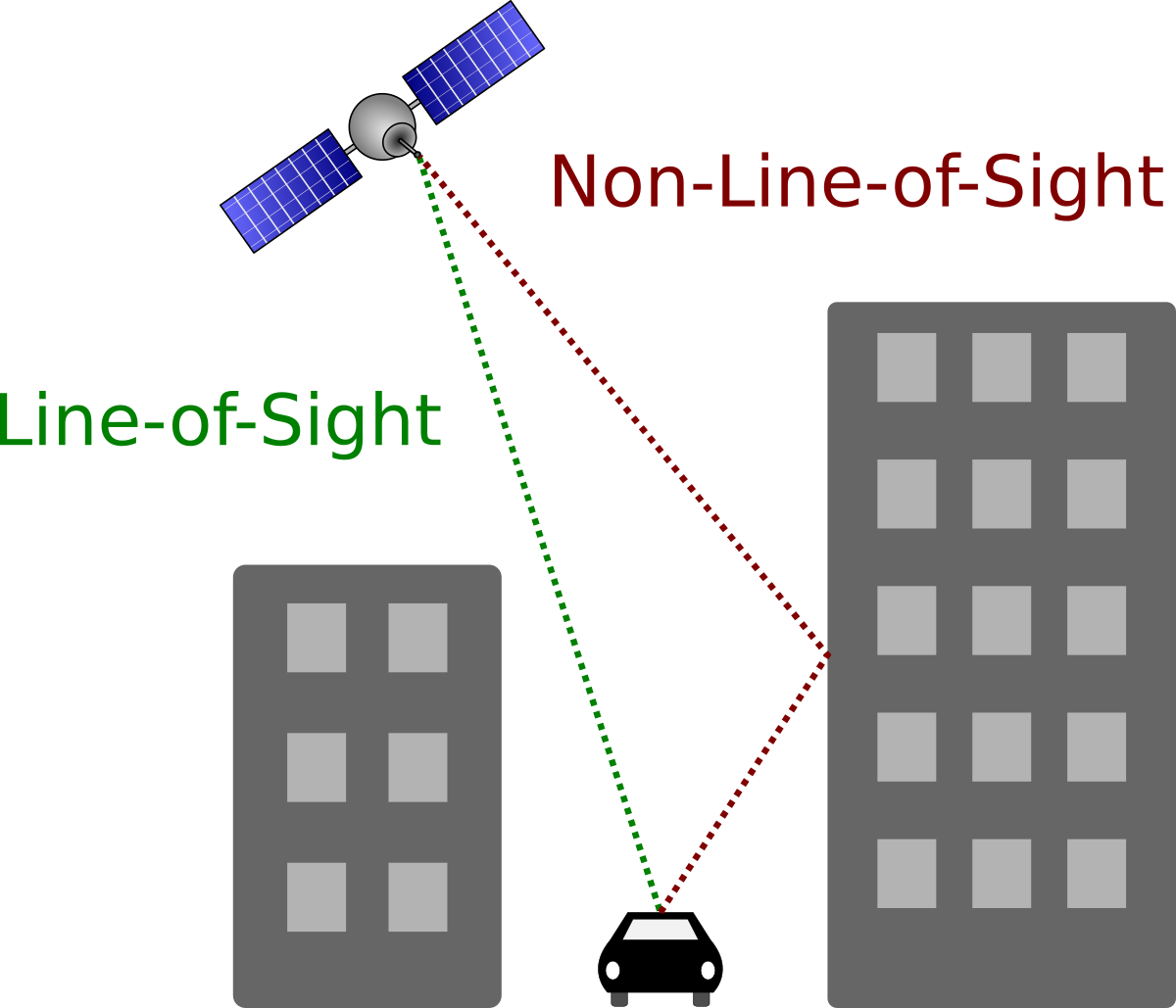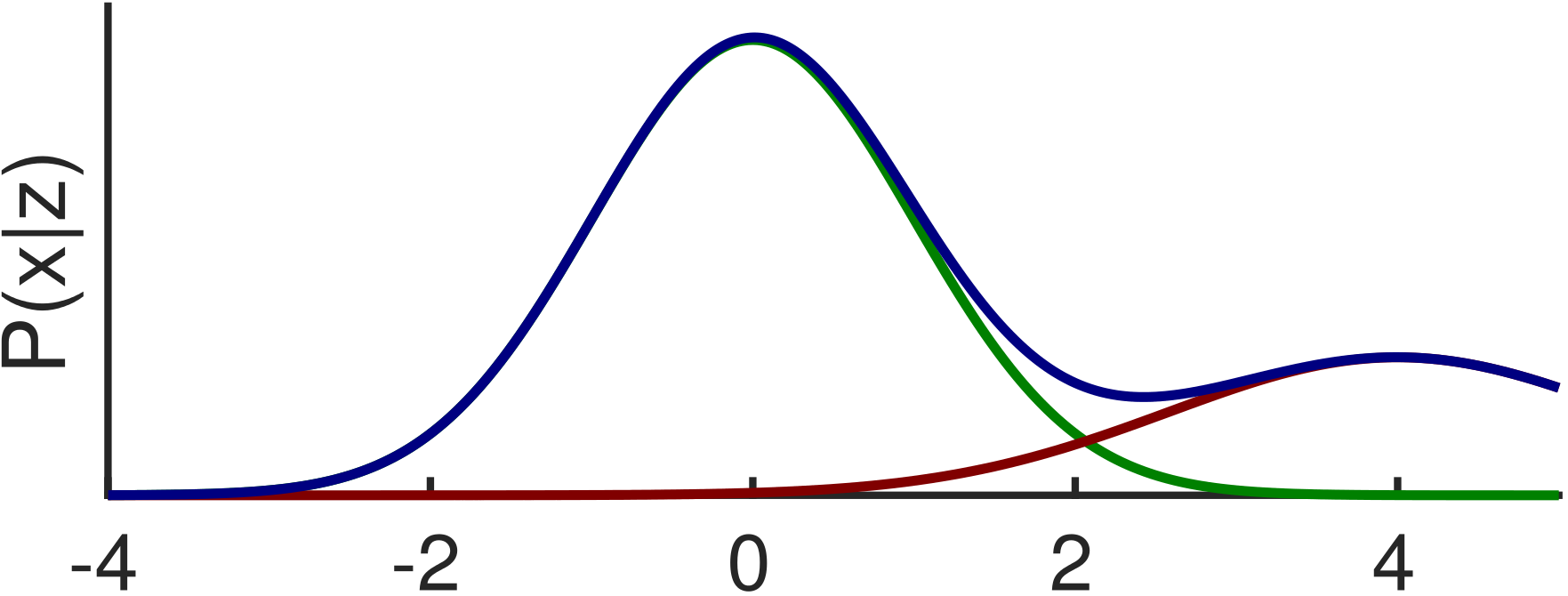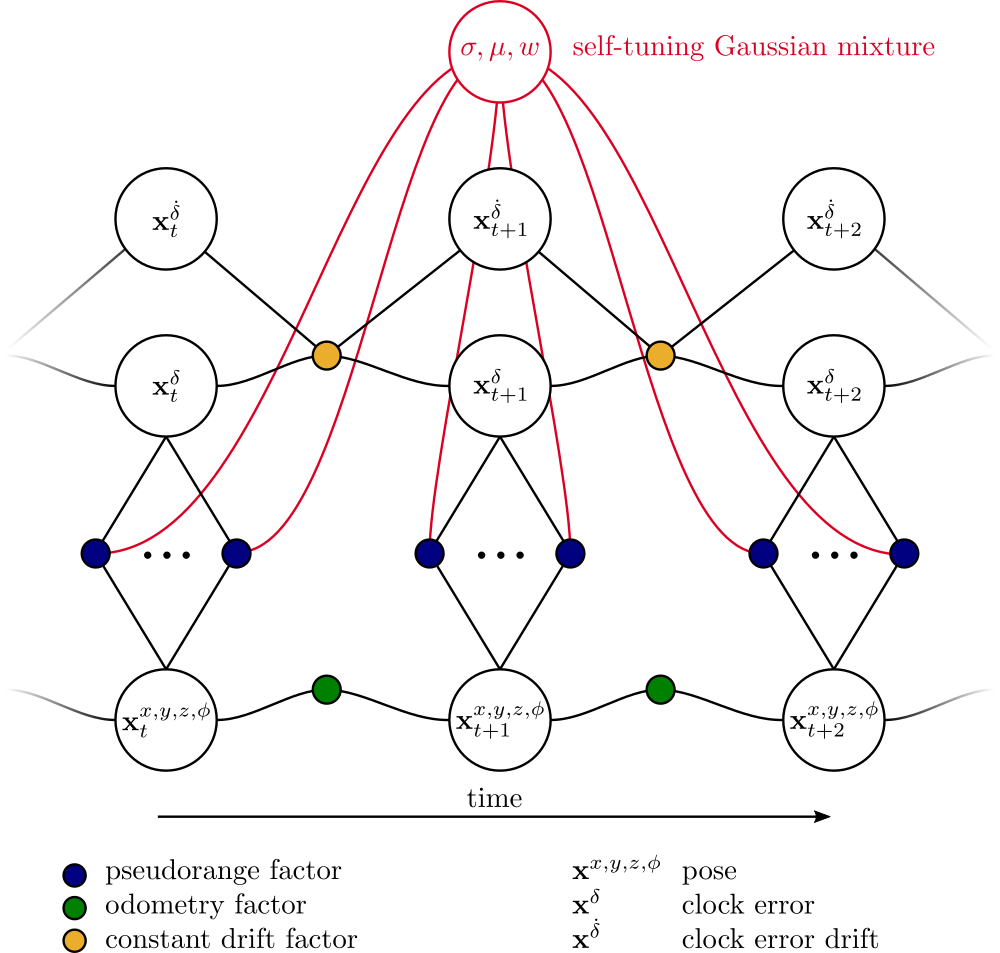Automation Technology
Self-tuning Mixtures

# Self-tuning Mixtures for Robust Factor Graph Optimization## The Problem of non-Gaussian Errors

To estimate the current state of a robotic or autonomous system, almost every probabilistic sensor fusion algorithm is constructed by assuming specific error properties of one or multiple sensors. Regardless of the concrete choice of the model, a violation of model assumptions can have a strong impact on the estimation quality. Such non-Gaussian errors can have a variety of reasons. Wheel odometry can slip and return too high values and ultrasonic range finders or radar can measure signals that are reflected from multiple surfaces. Radio-based ranging like distance measurements or satellite-based localization can suffer from non-line-of-sight (NLOS) Effect. One example is shown on the right, where the NLOS reflection causes a multimodal Sum-of-Gaussian distribution. More Information: NLOS in GNSS systemsSince the resulting distributions are asymmetric or heavy-tailed, algorithms like Kalman filters or factor graphs are not able to estimate the systems state in a reliable way. Instead the estimation result will be strongly biased. There already exist approaches to use non-Gaussian distributions in factor graphs. However, their common problem is the lack of concepts to parametrize this distributions without prior knowledge that is often not available.

## Self-tuning MixturesWe recently introduced the concept of Self-tuning Gaussian mixture models (GMM). The proposed algorithm combines a efficient approximation of a GMM with an implicit Expectation-Maximization algorithm to tune the parameter of the GMM. As shown on the right, we include the error models' parameter directly in the estimation problem that is visualized as factor graph. We solve this problem jointly by applying non-linear least-squares optimization optimization.

Since the initial parametrization of the GMM is quite tricky, we recently proposed an improved version of this algorithm: Adaptive Mixtures.

The evaluation on a GNSS localization dataset showed how the dynamic estimation of the error model can improve the overall estimation quality. The Dataset itself is online available.